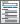Home  /  Products  /  Features  /  Binary, count, and limited outcomes

## Binary, fractional, count, and limited outcomes

Is your response binary (for example, employed or unemployed), ordinal (education level), count (number of children), or censored (ticket sales in an existing venue)? Stata has maximum likelihood estimators—logistic, probit, ordered probit, multinomial logit, Poisson, tobit, and many others—that estimate the relationship between such outcomes and their determinants. A vast array of tools is available to analyze such models. Predict outcomes and their confidence intervals. Test equality of parameters or any linear or nonlinear combination of parameters. And much more.

Tobit/censored regressionTruncated regressionInterval regressionPoisson and negative binomial regression• Left, right, and interval censoring
• Robust, cluster–robust, bootstrap, and jackknife standard errors
• Linear constraints

Zero-inflated count models

Truncated count models

Probit regression

Watch Probit regression tutorials

Sample-selection models for continuous outcomesSample selection with a binary outcomeStereotype logistic regression• Additive models of relative risk
• Relative excess risk due to interaction (RERI) statistic
• Attributable proportion (AP)
• Synergy index (SI)
• Supports binomial generalized linear models; logistic, Poisson, and negative binomial regressions; and Cox and other survival models

• View and run all postestimation features for your command
• Automatically updated as estimation commands are run
• Automatically create indicators based on categorical variables
• Form interactions among discrete and continuous variables
• Include polynomial terms
• Perform contrasts of categories/levels
Watch Introduction to margins in Stata tutorials
Watch Profile plots and interaction plots in Stata tutorials